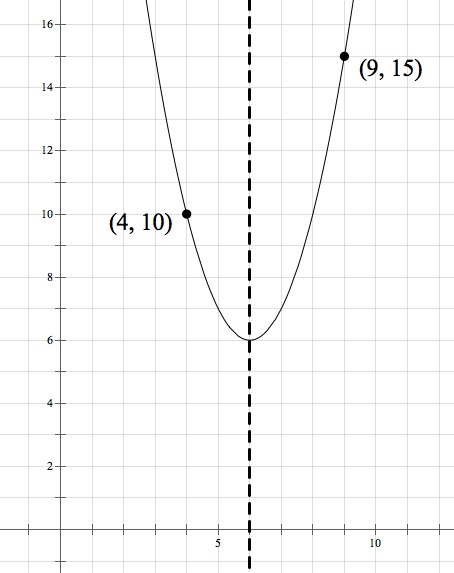Hi, Mike! Can you explain the second way we can approach question number 6 from the Parabola chapter? (The two points where the higher y-coordinate is also farther from the line of symmetry.) It would be great if you can provide an example. Thank you.

Sure. Here’s the question:The key, as I say in the solution, is to look for points that are consistent with what you know about the parabola given the equation: that it opens upwards and that its line of symmetry is at x = 6. Because parabolas get wider and wider, points in a parabola that opens up must get higher and higher the farther they get from the line of symmetry.

Therefore, you’re looking for either

1. Two points with the same y-coordinate that are the same distance from the line of symmetry, or
2. Two points that are otherwise consistent with the shape of an upwards-opening parabola that’s symmetrical about x = 6.

Choice D does the first one—x = 2 and x = 10 are each 4 away from the line of symmetry at x = 6, and both points have the same y-coordinate of –5.

No choice satisfies the second one (otherwise there’d be more than one possible right answer!) but a choice like (4, 10) and (9, 15) would. (4, 10) is closer to x = 6 and has a lower y-value; (9, 15) is farther from x = 6 and has a higher y-value: